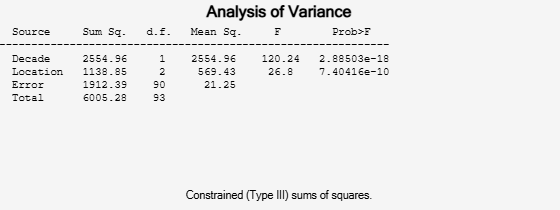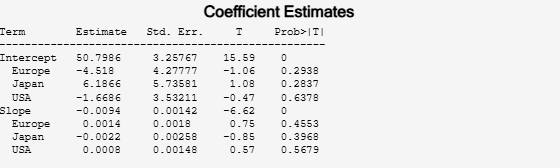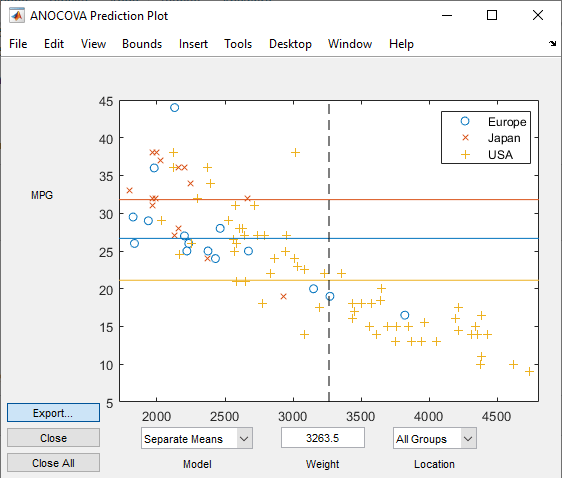# Test Differences Between Category Means

This example shows how to test for significant differences between category (group) means using a t-test, two-way ANOVA (analysis of variance), and ANOCOVA (analysis of covariance) analysis.

Determine if the expected miles per gallon for a car depends on the decade in which it was manufactured or the location where it was manufactured.

```load carsmall unique(Model_Year)```
```ans = 3×1 70 76 82 ```

The variable `MPG` has miles per gallon measurements on a sample of 100 cars. The variables `Model_Year` and `Origin` contain the model year and country of origin for each car.

The first factor of interest is the decade of manufacture. There are three manufacturing years in the data.

### Create Factor for Decade of Manufacture

Create a categorical array named `Decade` by merging the observations from years `70` and `76` into a category labeled `1970s`, and putting the observations from `82` into a category labeled `1980s`.

```Decade = discretize(Model_Year,[70 77 82], ... "categorical",["1970s","1980s"]); categories(Decade)```
```ans = 2x1 cell {'1970s'} {'1980s'} ```

### Plot Data Grouped by Category

Draw a box plot of miles per gallon, grouped by the decade of manufacture.

```boxplot(MPG,Decade) title("Miles per Gallon, Grouped by Decade of Manufacture")```The box plot suggests that miles per gallon is higher in cars manufactured during the 1980s compared to the 1970s.

### Compute Summary Statistics

Compute the mean and variance of miles per gallon for each decade.

`[xbar,s2,grp] = grpstats(MPG,Decade,["mean","var","gname"])`
```xbar = 2×1 19.7857 31.7097 ```
```s2 = 2×1 35.1429 29.0796 ```
```grp = 2x1 cell {'1970s'} {'1980s'} ```

This output shows that the mean miles per gallon in the 1980s was approximately `31.71`, compared to `19.79` in the 1970s. The variances in the two groups are similar.

### Conduct Two-Sample t-Test for Equal Group Means

Conduct a two-sample t-test, assuming equal variances, to test for a significant difference between the group means. The hypothesis is

`$\begin{array}{l}{H}_{0}:{\mu }_{70}={\mu }_{80}\\ {H}_{A}:{\mu }_{70}\ne {\mu }_{80}.\end{array}$`

```MPG70 = MPG(Decade=="1970s"); MPG80 = MPG(Decade=="1980s"); [h,p] = ttest2(MPG70,MPG80)```
```h = 1 ```
```p = 3.4809e-15 ```

The logical value `1` indicates the null hypothesis is rejected at the default 0.05 significance level. The p-value for the test is very small. There is sufficient evidence that the mean miles per gallon in the 1980s differs from the mean miles per gallon in the 1970s.

### Create Factor for Location of Manufacture

The second factor of interest is the location of manufacture. First, convert `Origin` to a categorical array.

```Location = categorical(cellstr(Origin)); tabulate(Location)```
``` Value Count Percent France 4 4.00% Germany 9 9.00% Italy 1 1.00% Japan 15 15.00% Sweden 2 2.00% USA 69 69.00% ```

There are six different countries of manufacture. The European countries have relatively few observations.

### Merge Categories

Combine the categories `France`, `Germany`, `Italy`, and `Sweden` into a new category named `Europe`.

```Location = mergecats(Location, ... ["France","Germany","Italy","Sweden"],"Europe"); tabulate(Location)```
``` Value Count Percent Europe 16 16.00% Japan 15 15.00% USA 69 69.00% ```

### Compute Summary Statistics

Compute the mean miles per gallon, grouped by the location of manufacture.

`[meanMPG,locationGroup] = grpstats(MPG,Location,["mean","gname"])`
```meanMPG = 3×1 26.6667 31.8000 21.1328 ```
```locationGroup = 3x1 cell {'Europe'} {'Japan' } {'USA' } ```

This result shows that average miles per gallon is lowest for the sample of cars manufactured in the U.S.

### Conduct Two-Way ANOVA

Conduct a two-way ANOVA to test for differences in expected miles per gallon between factor levels for `Decade` and `Location`.

The statistical model is

`$MP{G}_{ij}=\mu +{\alpha }_{i}+{\beta }_{j}+{ϵ}_{ij},\phantom{\rule{1em}{0ex}}i=1,2;\phantom{\rule{0.5em}{0ex}}j=1,2,3,$`

where $MP{G}_{ij}$ is the response, miles per gallon, for cars made in decade $i$ at location $j$. The treatment effects for the first factor, decade of manufacture, are the ${\alpha }_{i}$ terms (constrained to sum to zero). The treatment effects for the second factor, location of manufacture, are the ${\beta }_{j}$ terms (constrained to sum to zero). The ${ϵ}_{ij}$ are uncorrelated, normally distributed noise terms.

The hypotheses to test are equality of decade effects,

`$\begin{array}{l}{H}_{0}:{\alpha }_{1}={\alpha }_{2}=0\\ {H}_{A}:at\phantom{\rule{0.2222222222222222em}{0ex}}least\phantom{\rule{0.2222222222222222em}{0ex}}one\phantom{\rule{0.2222222222222222em}{0ex}}{\alpha }_{i}\ne 0,\end{array}$`

and equality of location effects,

`$\begin{array}{l}{H}_{0}:{\beta }_{1}={\beta }_{2}={\beta }_{3}=0\\ {H}_{A}:at\phantom{\rule{0.2222222222222222em}{0ex}}least\phantom{\rule{0.2222222222222222em}{0ex}}one\phantom{\rule{0.2222222222222222em}{0ex}}{\beta }_{j}\ne 0.\end{array}$`

You can conduct a multiple-factor ANOVA using `anovan`.

```anovan(MPG,{Decade,Location}, ... "Varnames",["Decade","Location"]);```This output shows the results of the two-way ANOVA. The p-value for testing the equality of decade effects is `2.88503e-18`, so the null hypothesis is rejected at the 0.05 significance level. The p-value for testing the equality of location effects is `7.40416e-10`, so this null hypothesis is also rejected.

### Conduct ANOCOVA Analysis

A potential confounder in this analysis is car weight. Cars with greater weight are expected to have lower gas mileage. Include the variable `Weight` as a continuous covariate in the ANOVA; that is, conduct an ANOCOVA analysis.

Assuming parallel lines, the statistical model is

`$MP{G}_{ijk}=\mu +{\alpha }_{i}+{\beta }_{j}+\gamma Weigh{t}_{ijk}+{ϵ}_{ijk},\phantom{\rule{1em}{0ex}}i=1,2;\phantom{\rule{0.5em}{0ex}}j=1,2,3;\phantom{\rule{0.5em}{0ex}}k=1,...,100.$`

The difference between this model and the two-way ANOVA model is the inclusion of the continuous predictor $Weigh{t}_{ijk}$, the weight for the $k$th car, which was made in the $i$th decade and in the$j$th location. The slope parameter is $\gamma$.

Add the continuous covariate as a third group in the second `anovan` input argument. Use the `Continuous` name-value argument to specify that `Weight` (the third group) is continuous.

```anovan(MPG,{Decade,Location,Weight},"Continuous",3, ... "Varnames",["Decade","Location","Weight"]);```This output shows that when car weight is considered, there is insufficient evidence of a manufacturing location effect (p-value = `0.1044`).

### Use Interactive Tool

You can use the interactive `aoctool` to explore this result. This command opens three dialog boxes.

`aoctool(Weight,MPG,Location);`In the ANOCOVA Prediction Plot dialog box, select the Separate Means model.This output shows that when you do not include `Weight` in the model, there are fairly large differences in the expected miles per gallon among the three manufacturing locations. Note that here the model does not adjust for the decade of manufacturing.

Now, select the Parallel Lines model.When you include `Weight` in the model, the difference in expected miles per gallon among the three manufacturing locations is much smaller.